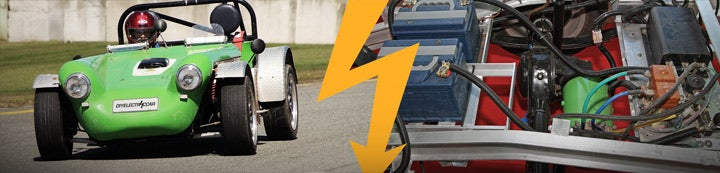1 - 3 of 3 Posts

#### DrGee

·
##### Registered
Joined
·
152 Posts
Discussion Starter · ·
I found these formulae and worked examples on some technical site. I found them to be extremely useful.
Using formulae (1) + (2), I was able to estimate the total forces acting on the car against it's forward motion (on level ground).
I worked out that a 2 ton Jeep Grand Cherokee with a 0.41 coefficient of drag, required 60 kW to maintain 75 mph on level ground, if my memory serves me right.
I also put in the numbers for a Tesla Model S to test the formulae. I got about 20kw to maintain 65mph on level ground. That gives the 100 Kwh Model S a range of 325 miles at 65mph. This is close to the figures Tesla advertises, so I suppose that validates the formulae.
I tried to attach the whole document, but it's a pdf and it didn't attach.

(1) Aerodynamic drag resistance-
Fd = cd 1/2 ρ v2 A

where

Fd = drag force (N)

cd = drag coefficient

ρ = density of fluid (1.2 kg/m3 for air at NTP)

v = flow velocity (m/s)

A = characteristic frontal area of the body (m2)

(2)The rolling resistance can be expressed as
Fr = c W
where

Fr = rolling resistance or rolling friction (N, lbf)

c = rolling resistance coefficient - dimensionless (coefficient of rolling friction - CRF)

W = m ag

= normal force - or weight - of the body (N, lbf)

m = mass of body (kg, lb)

ag = acceleration of gravity (9.81 m/s2, 32.174 ft/s2

Power Required
power from an engine to keep a car at constant speed can be calculated as
P = FT v / η
where P = engine power (in Watts)
FT = total forces acting on the car - rolling resistance force , gradient resistance force and aerodynamic drag resistance (in Newtons )
v = velocity of the car (m/s)
η = overall efficiency in the transmission, normally ranging 0.85 (low gear) - 0.9 (direct drive)
For a car that accelerates the acceleration force and required Engine Power must be added to the total force.
The required engine power for a car driving on a flat surface with constant speed 90 km/h with an aerodynamic resistance force 250 N and rolling resistance force N and overall efficiency 0.85 - can be calculated as P = ((250 N) + (400 N)) (90 km/h) (1000 m/km) (1/3600 h/s) / 0.85 = 19118 W = 19 kW

1 - 3 of 3 Posts